# Project: Sequences of Circles, Line Segments, PolygonsA sequence of polygons

For this project you need to create your own sequence diagrams, and figure out a given sequence by drawing by hand before using the command on GeoGebra.

Page 1: Title, Name, Date, Introduction;

Page 2: Your own sequence of circles screenshot with command and explanation;

Page 3: Your own sequence of line segments screenshot with command and explanation;

Page 4: A hand drawn diagram of a given command (from teacher) for a sequence of circles; the GeoGebra diagram and description of errors/accuracies.

Page 5: A hand drawn diagram of a given command (from teacher) for a sequence of line segments; the GeoGebra diagram and description of errors/accuracies.

Before beginning, become acquainted with the various GeoGebra commands by following these instructions:

To begin, open a GeoGebra classic page, (close the keyboard) then open a long input bar at the bottom as follows:For each command given as an example, either type carefully to include all brackets or copy and paste into the input bar on the GeoGebra page.

# A Sequence

A sequence until now has simply been a list of numbers. The sequence command in GeoGebra is as follows: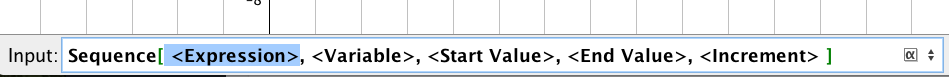Fill in the input options as follows: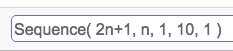Sequence(2n+1,n,1,10,1)

Press enter, to generate a simple sequence of numbers:# Sequences of Circles

The circle command requires a point for the center, and a radius.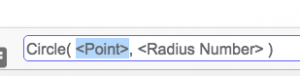Example 1. Clear your GeoGebra screen and type in the following set of circles, one at a time:

Circle((1,1),1)

Circle((2,2),1)

Circle((3,3),1)

Circle((4,4),1)

This should give four circles, all with different centers but radius 1.

What they have in common is that the centers are at the point (n,n) betweenandNow, clear the screen and enter all of them together with one sequence command:Sequence(circle((n,n),1),n,1,4,1)

This means, circle center (n,n) with radius 1, for all values of n between 1 and 4, going up in ones.

Example 2.

Now enter

Sequence(circle((n,n),1),n,1,4,0.1)

Which means, circle center (n,n) with radius 1, for all values of n between 1 and 4, going up in 0.1s – which draws a total of 31 circles!

Example 3. Suppose we make a sequence of circles with centerand with radius.

Try out the following command: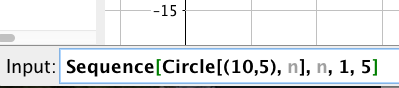Sequence[Circle[(10,5),n],n,1,5]

Example 4. Now let’s add the ‘increment parameter’ and make it 0.1, to draw many more circles.

Sequence[Circle[(10,5), n], n, 1, 5, 0.1]

The final parameter changes the increment offrom the default value 1 to 0.1. More steps between 1 and 5 – more circles.

## Make some of your own

1. Make a sequence where the center of the circle is constant, but the radius changes.
2. Make a sequence where the center of the circle changes, but the radius is constant.
3. Make a sequence where the center and the radius both change.

## Figure out the sequence command

Now see if you can reproduce the following diagrams:

### TUnnel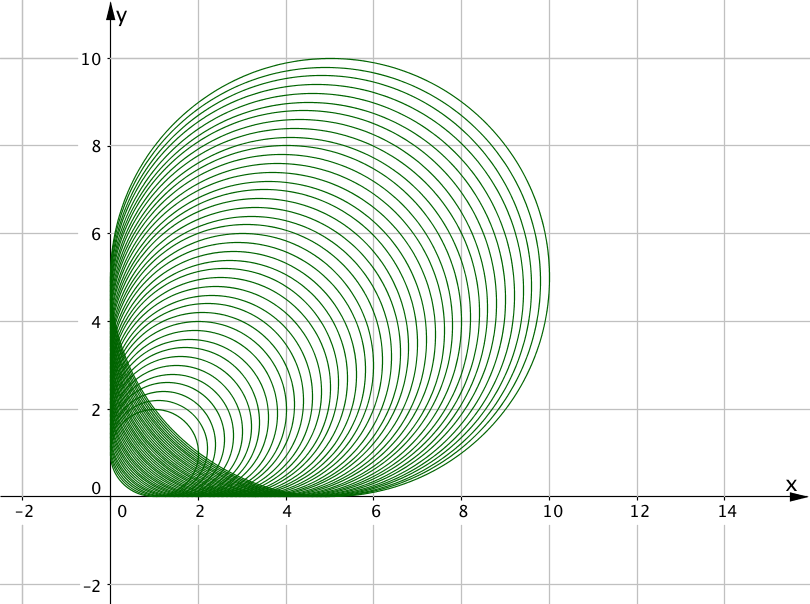### eye 1### eye 2# Sequences of Line Segments

The following diagram uses the sequence command along with the command Segment[<point>,<point>] to create a sequence of line segments.

Example 1. What will the following command produce? Try it out.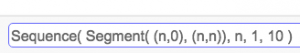Example 2. Observe the start/end point to a few of line segments to see the pattern of start and end points.